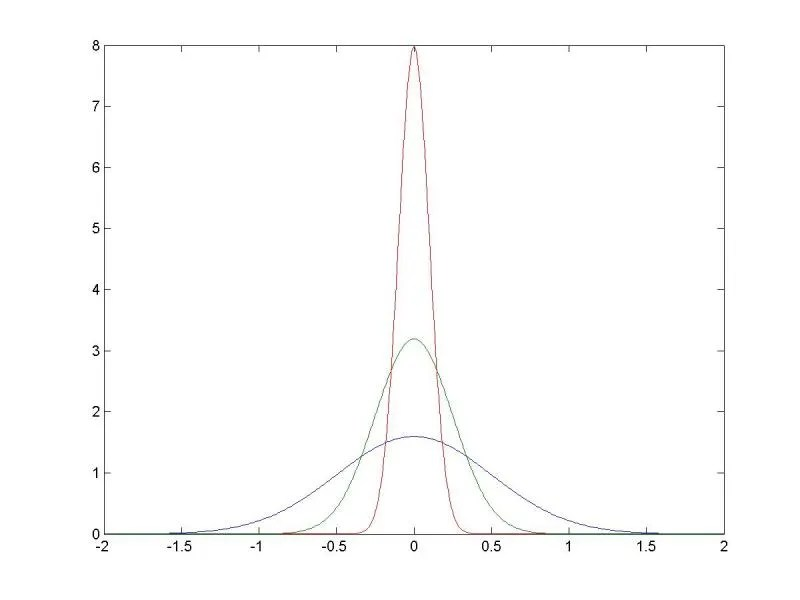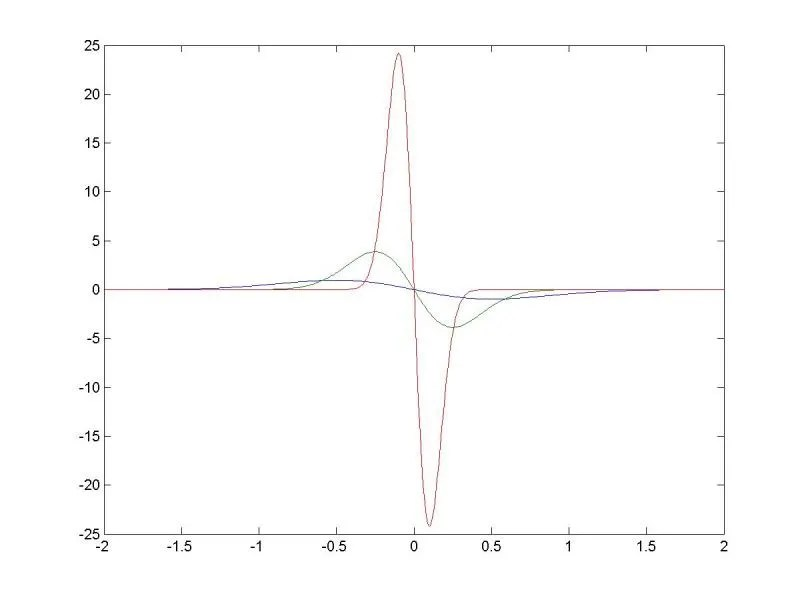College Math Teaching

January 7, 2011

The Dirac Delta Function in an Elementary Differential Equations Course

The Dirac Delta Function in Differential Equations

The delta ”function” is often introduced into differential equations courses during the section on Laplace transforms. Of course the delta
”function” isn’t a function at all but rather what is known as a ”distribution” (more on this later)

A typical introduction is as follows: if one is working in classical mechanics and one applies a force$F(t)$ to a constant mass$m$ at time$t,$ then one can define the impulse$I$ of$F$ over an interval$[a,b]$ by$I=\int_{a}^{b}F(t)dt=m(v(a)-v(b))$ where$v$ is the velocity. So we can do a translation to set$a=0$ and then consider a unit impulse and vary$F(t)$
according to where$b$ is; that is, define$\delta ^{\varepsilon}(t)=\left\{ \begin{array}{c}\frac{1}{\varepsilon },0\leq t\leq \varepsilon \\ 0\text{ elsewhere}\end{array}\right. .$

Then$F(t)=\delta ^{\varepsilon }(t)$ is the force function that produces unit impulse for a given$\varepsilon >0.$

Then we wave our hands and say$\delta (t)=\lim _{\varepsilon \rightarrow 0}\delta ^{\varepsilon }(t)$ (this is a great reason to introduce the concept of the limit of functions in a later course) and then argue that for all functions that are continuous over an interval containing 0,$\int_{0}^{\infty }\delta (t)f(t)dt=f(0)$.

The (hand waving) argument at this stage goes something like: ”the mean value theorem for integrals says that there is a$c_{\varepsilon }$
between$0$ and$\varepsilon$ such that$\int_{0}^{\varepsilon }\delta^{\varepsilon }(t)f(t)dt=\frac{1}{\varepsilon}f(c_{\varepsilon})(\varepsilon -0)=f(c_{\varepsilon })$ Therefore as$\varepsilon\rightarrow 0,$$\int_{0}^{\varepsilon }\delta^{\varepsilon}(t)f(t)dt=f(c_{\varepsilon })\rightarrow f(0)$ by continuity. Therefore we can define the Laplace transform$L(\delta (t))=e^{-s0}=1.$

Illustrating what the delta ”function” does.

I came across this example by accident; I was holding a review session for students and asked for them to give me a problem to solve.

They chose$y^{\prime \prime }+ay^{\prime }+by=\delta$ (I can remember what$a$ and$b$ were but they aren’t important here as we will see) with initial conditions$y(0)=0,y^{\prime }(0)=-1$

So using the Laplace transform, we obtained:$(s^{2}+as+b)Y-sy(0)-y^{\prime }(0)-ay(0)=1$

But with$y(0)=0,y^{\prime }(0)=-1$ this reduces to$(s^{2}+as+b)Y+1=1\rightarrow Y=0$

In other words, we have the ”same solution” as if we had$y^{\prime\prime }+ay^{\prime }+by=0$ with$y(0)=0,y^{\prime }(0)=0$.

So that might be a way to talk about the delta ”function”; it is exactly the ”impulse” one needs to ”cancel out” an initial velocity of$-1$ or,
equivalently, to give an initial velocity of$1$ and to do so instantly.

Another approach to the delta function

Though it is true that$\int_{-\infty }^{\infty }\delta^{\varepsilon }(t)dt=1$ for all$\varepsilon$ and$\int_{-\infty}^{\infty }\delta (t)dt=1$ by design, note that$\delta ^{\varepsilon }(t)$fails to be continuous at$0$ and at$\varepsilon$.

So, can we obtain the delta ”function” as a limit of other functions that are everywhere continuous and differentiable?

In an attempt to find such a family of functions, It is a fun exercise to look at a limit of normal density functions with mean zero:$f_{\sigma }(t)=\frac{1}{\sigma \sqrt{2\pi }}\exp (-\frac{1}{2\sigma ^{2}}t^{2})$. Clearly for all$\sigma >0,\int_{-\infty }^{\infty }f_{\sigma}(t)dt=1$ and$\int_{0}^{\infty }f_{\sigma }(t)dt=\frac{1}{2}$.

Here is the graph of some of these functions: we use$\sigma = .5$,$\sigma = .25$ and$\sigma = .1$ respectively.Calculating the Laplace transform$L(\frac{1}{\sigma \sqrt{2\pi }}\exp (-\frac{1}{2\sigma ^{2}}t^{2}))=$$\frac{1}{\sigma \sqrt{2\pi }}\int_{0}^{\infty }\exp (-\frac{1}{2\sigma^{2}}t^{2})\exp (-st)dt=$

Do some algebra to combine the exponentials, complete the square and do some algebra to obtain:$\frac{1}{\sigma \sqrt{2\pi }}\int_{0}^{\infty }\exp (-\frac{1}{2\sigma ^{2}}(t+\sigma ^{2}s)^{2})\exp (\frac{s^{2}\sigma^{2}}{2})dt=\exp (\frac{s^{2}\sigma ^{2}}{2})[\frac{1}{\sigma \sqrt{2\pi }}\int_{0}^{\infty }\exp (-\frac{1}{2\sigma ^{2}}(t+\sigma^{2}s)^{2})dt]$

Now do the usual transformation to the standard normal random variable via$z=\dfrac{t+\sigma ^{2}s}{\sigma }$

And we obtain:$L(f_{\sigma }(t))=\exp (\frac{s^{2}\sigma ^{2}}{2})P(Z>\sigma s)$ for all$\sigma >0$. Note: assume$s>0$ and that$P$ is shorthand for the usual probability distribution function.

Now if we take a limit as$\sigma \rightarrow 0$ we get$\frac{1}{2}$ on the right hand side.

Hence, one way to define$\delta$ is as$2\lim _{\sigma \rightarrow0}f_{\sigma }(t)$ . This means that while$\lim_{\sigma \rightarrow0}\int_{-\infty }^{\infty }2f_{\sigma }(t)dt$ is off by a factor of 2,$\lim_{\sigma \rightarrow 0}\int_{0}^{\infty }2f_{\sigma }(t)dt=1$ as desired.

Since we now have derivatives of the functions to examine, why don’t we?$\frac{d}{dt}2f_{\sigma }(t)=-\frac{2t}{\sigma ^{3}\sqrt{2\pi }}\exp (-\frac{1}{2\sigma ^{2}}t^{2})$ which is zero at$t=0$ for all$\sigma >0.$ But the behavior of the derivative is interesting: the derivative is at its minimum at$t=\sigma$ and at its maximum at$t=-\sigma$ (as we tell our probability students: the standard deviation is the distance from the origin to the inflection points) and as$\sigma \rightarrow 0,$ the inflection points get closer together and the second derivative at the
origin approaches$-\infty ,$ which can be thought of as an instant drop from a positive velocity at$t=0$.

Here are the graphs of the derivatives of the density functions that were plotted above; note how the part of the graph through the origin becomes more vertical as the standard deviation approaches zero.